Documentation

### This is machine translation

Mouseover text to see original. Click the button below to return to the English version of the page.

## Modelling Tail Data with the Generalized Pareto Distribution

This example shows how to fit tail data to the Generalized Pareto distribution by maximum likelihood estimation.

Fitting a parametric distribution to data sometimes results in a model that agrees well with the data in high density regions, but poorly in areas of low density. For unimodal distributions, such as the normal or Student's t, these low density regions are known as the "tails" of the distribution. One reason why a model might fit poorly in the tails is that by definition, there are fewer data in the tails on which to base a choice of model, and so models are often chosen based on their ability to fit data near the mode. Another reason might be that the distribution of real data is often more complicated than the usual parametric models.

However, in many applications, fitting the data in the tail is the main concern. The Generalized Pareto distribution (GP) was developed as a distribution that can model tails of a wide variety of distributions, based on theoretical arguments. One approach to distribution fitting that involves the GP is to use a non-parametric fit (the empirical cumulative distribution function, for example) in regions where there are many observations, and to fit the GP to the tail(s) of the data.

### The Generalized Pareto Distribution

The Generalized Pareto (GP) is a right-skewed distribution, parameterized with a shape parameter, k, and a scale parameter, sigma. k is also known as the "tail index" parameter, and can be positive, zero, or negative.

```x = linspace(0,10,1000); plot(x,gppdf(x,-.4,1),'-', x,gppdf(x,0,1),'-', x,gppdf(x,2,1),'-'); xlabel('x / sigma'); ylabel('Probability density'); legend({'k < 0' 'k = 0' 'k > 0'}); ```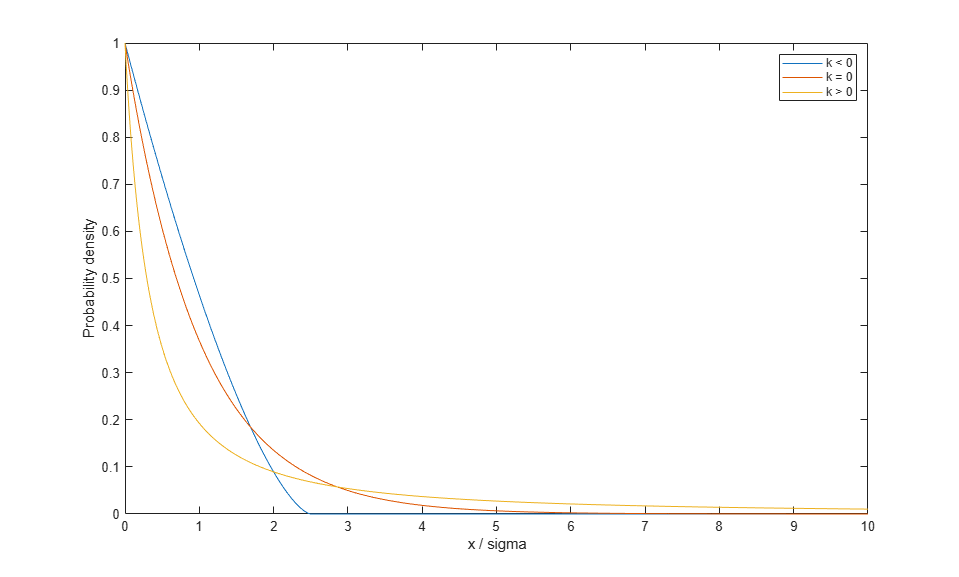Notice that for k < 0, the GP has zero probability above an upper limit of -(1/k). For k >= 0, the GP has no upper limit. Also, the GP is often used in conjunction with a third, threshold parameter that shifts the lower limit away from zero. We will not need that generality here.

The GP distribution is a generalization of both the exponential distribution (k = 0) and the Pareto distribution (k > 0). The GP includes those two distributions in a larger family so that a continuous range of shapes is possible.

### Simulating Exceedance Data

The GP distribution can be defined constructively in terms of exceedances. Starting with a probability distribution whose right tail drops off to zero, such as the normal, we can sample random values independently from that distribution. If we fix a threshold value, throw out all the values that are below the threshold, and subtract the threshold off of the values that are not thrown out, the result is known as exceedances. The distribution of the exceedances is approximately a GP. Similarly, we can set a threshold in the left tail of a distribution, and ignore all values above that threshold. The threshold must be far enough out in the tail of the original distribution for the approximation to be reasonable.

The original distribution determines the shape parameter, k, of the resulting GP distribution. Distributions whose tails fall off as a polynomial, such as Student's t, lead to a positive shape parameter. Distributions whose tails decrease exponentially, such as the normal, correspond to a zero shape parameter. Distributions with finite tails, such as the beta, correspond to a negative shape parameter.

Real-world applications for the GP distribution include modelling extremes of stock market returns, and modelling extreme floods. For this example, we'll use simulated data, generated from a Student's t distribution with 5 degrees of freedom. We'll take the largest 5% of 2000 observations from the t distribution, and then subtract off the 95% quantile to get exceedances.

```rng(3,'twister'); x = trnd(5,2000,1); q = quantile(x,.95); y = x(x>q) - q; n = numel(y) ```
```n = 100 ```

### Fitting the Distribution Using Maximum Likelihood

The GP distribution is defined for 0 < sigma, and -Inf < k < Inf. However, interpretation of the results of maximum likelihood estimation is problematic when k < -1/2. Fortunately, those cases correspond to fitting tails from distributions like the beta or triangular, and so will not present a problem here.

```paramEsts = gpfit(y); kHat = paramEsts(1) % Tail index parameter sigmaHat = paramEsts(2) % Scale parameter ```
```kHat = 0.0987 sigmaHat = 0.7156 ```

As might be expected, since the simulated data were generated using a t distribution, the estimate of k is positive.

### Checking the Fit Visually

To visually assess how good the fit is, we'll plot a scaled histogram of the tail data, overlayed with the density function of the GP that we've estimated. The histogram is scaled so that the bar heights times their width sum to 1.

```bins = 0:.25:7; h = bar(bins,histc(y,bins)/(length(y)*.25),'histc'); h.FaceColor = [.9 .9 .9]; ygrid = linspace(0,1.1*max(y),100); line(ygrid,gppdf(ygrid,kHat,sigmaHat)); xlim([0,6]); xlabel('Exceedance'); ylabel('Probability Density'); ```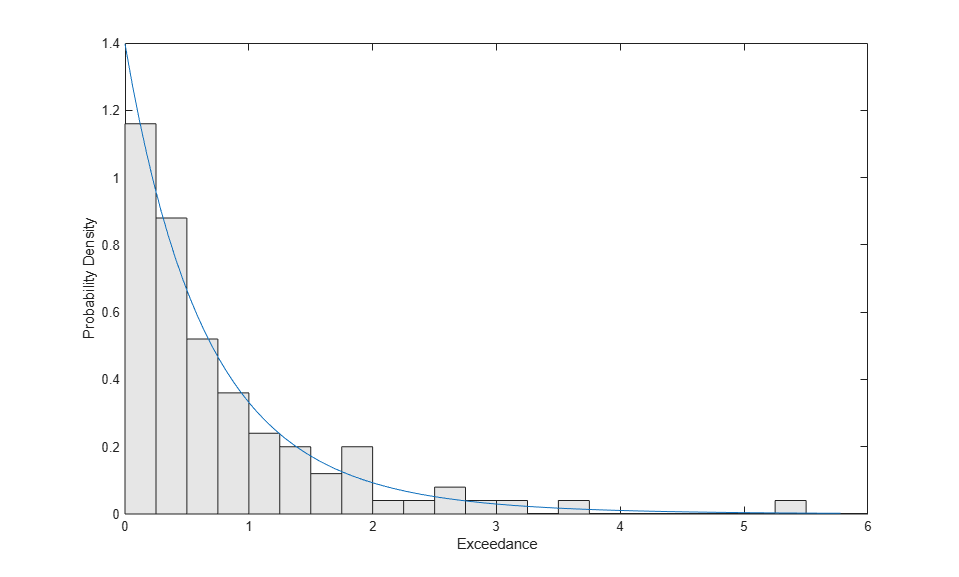We've used a fairly small bin width, so there is a good deal of noise in the histogram. Even so, the fitted density follows the shape of the data, and so the GP model seems to be a good choice.

We can also compare the empirical CDF to the fitted CDF.

```[F,yi] = ecdf(y); plot(yi,gpcdf(yi,kHat,sigmaHat),'-'); hold on; stairs(yi,F,'r'); hold off; legend('Fitted Generalized Pareto CDF','Empirical CDF','location','southeast'); ```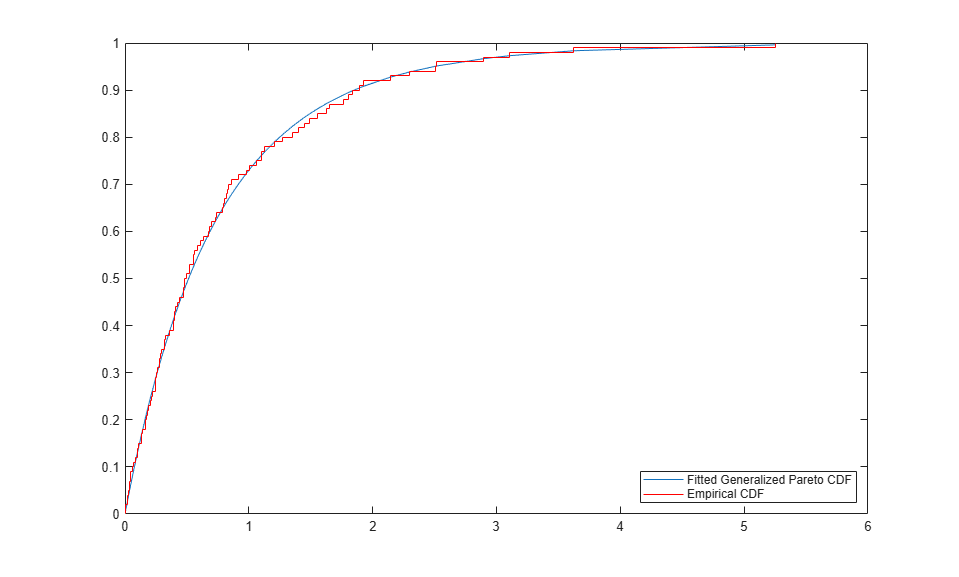### Computing Standard Errors for the Parameter Estimates

To quantify the precision of the estimates, we'll use standard errors computed from the asymptotic covariance matrix of the maximum likelihood estimators. The function `gplike` computes, as its second output, a numerical approximation to that covariance matrix. Alternatively, we could have called `gpfit` with two output arguments, and it would have returned confidence intervals for the parameters.

```[nll,acov] = gplike(paramEsts, y); stdErr = sqrt(diag(acov)) ```
```stdErr = 0.1158 0.1093 ```

These standard errors indicate that the relative precision of the estimate for k is quite a bit lower than that for sigma -- its standard error is on the order of the estimate itself. Shape parameters are often difficult to estimate. It's important to keep in mind that computation of these standard errors assumed that the GP model is correct, and that we have enough data for the asymptotic approximation to the covariance matrix to hold.

### Checking the Asymptotic Normality Assumption

Interpretation of the standard errors usually involves assuming that, if the same fit could be repeated many times on data that came from the same source, the maximum likelihood estimates of the parameters would approximately follow a normal distribution. For example, confidence intervals are often based this assumption.

However, that normal approximation may or may not be a good one. To assess how good it is in this example, we can use a bootstrap simulation. We will generate 1000 replicate datasets by resampling from the data, fit a GP distribution to each one, and save all the replicate estimates.

```replEsts = bootstrp(1000,@gpfit,y); ```

As a rough check on the sampling distribution of the parameter estimators, we can look at histograms of the bootstrap replicates.

```subplot(2,1,1); hist(replEsts(:,1)); title('Bootstrap estimates of k'); subplot(2,1,2); hist(replEsts(:,2)); title('Bootstrap estimates of sigma'); ```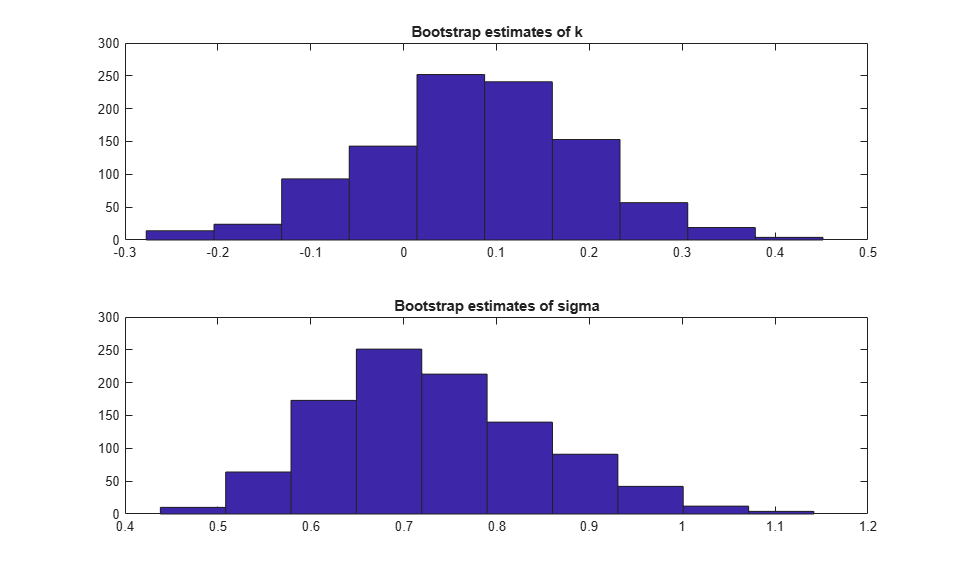### Using a Parameter Transformation

The histogram of the bootstrap estimates for k appears to be only a little asymmetric, while that for the estimates of sigma definitely appears skewed to the right. A common remedy for that skewness is to estimate the parameter and its standard error on the log scale, where a normal approximation may be more reasonable. A Q-Q plot is a better way to assess normality than a histogram, because non-normality shows up as points that do not approximately follow a straight line. Let's check that to see if the log transform for sigma is appropriate.

```subplot(1,2,1); qqplot(replEsts(:,1)); title('Bootstrap estimates of k'); subplot(1,2,2); qqplot(log(replEsts(:,2))); title('Bootstrap estimates of log(sigma)'); ```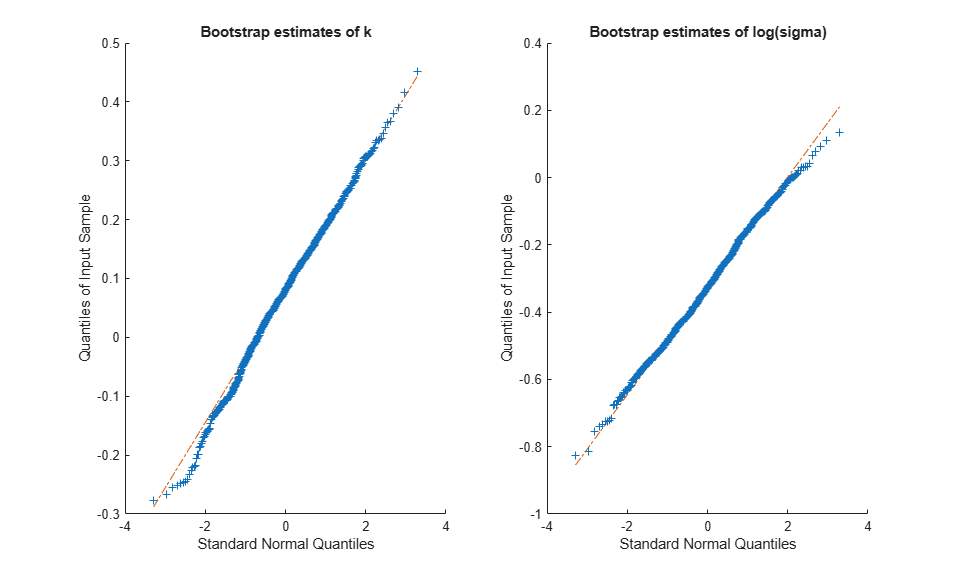The bootstrap estimates for k and log(sigma) appear acceptably close to normality. A Q-Q plot for the estimates of sigma, on the unlogged scale, would confirm the skewness that we've already seen in the histogram. Thus, it would be more reasonable to construct a confidence interval for sigma by first computing one for log(sigma) under the assumption of normality, and then exponentiating to transform that interval back to the original scale for sigma.

In fact, that's exactly what the function `gpfit` does behind the scenes.

```[paramEsts,paramCI] = gpfit(y); ```
```kHat kCI = paramCI(:,1) ```
```kHat = 0.0987 kCI = -0.1283 0.3258 ```
```sigmaHat sigmaCI = paramCI(:,2) ```
```sigmaHat = 0.7156 sigmaCI = 0.5305 0.9654 ```

Notice that while the 95% confidence interval for k is symmetric about the maximum likelihood estimate, the confidence interval for sigma is not. That's because it was created by transforming a symmetric CI for log(sigma).